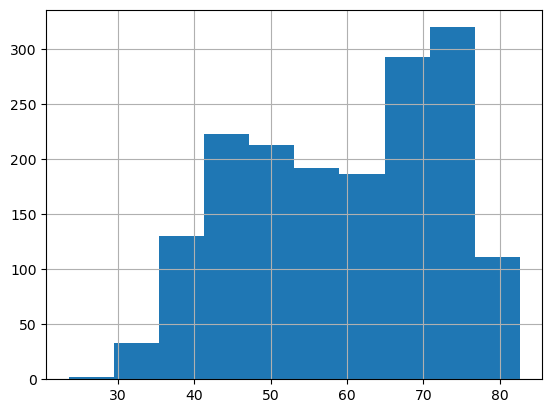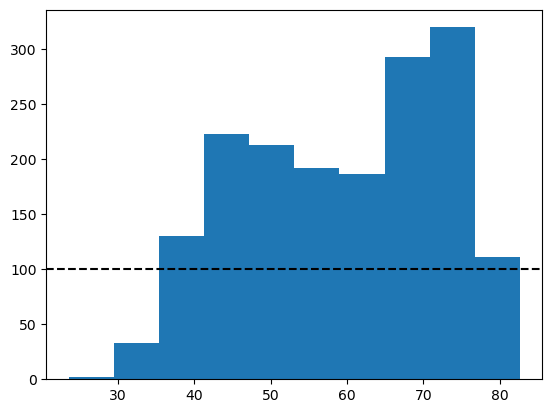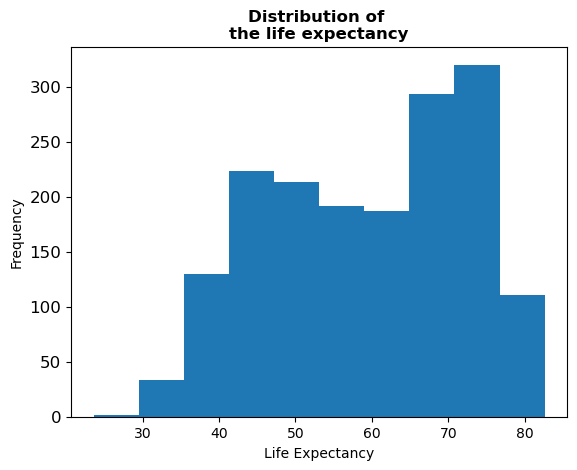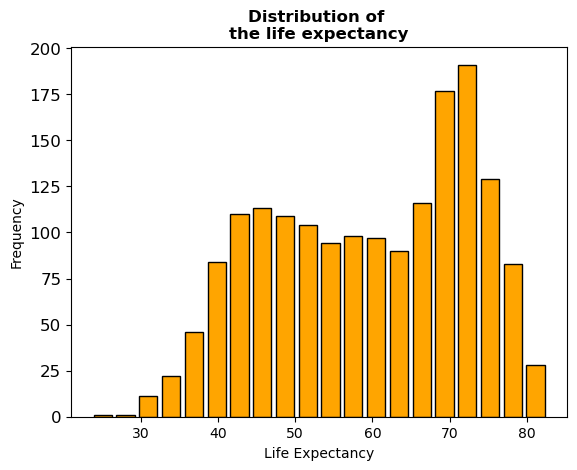## Libraries

Pandas is a popular open-source Python library used for data manipulation and analysis. It provides data structures and functions that make working with structured data, such as tabular data (like `Excel` spreadsheets or `SQL` tables), easy and intuitive.

To install Pandas, you can use the following command in your command-line interface (such as `Terminal` or `Command Prompt`):

`pip install pandas`

Matplotlib functionalities have been integrated into the pandas library, facilitating their use with `dataframes` and `series`. For this reason, you might also need to import the matplotlib library when building charts with Pandas.

This also means that they use the same functions, and if you already know Matplotlib, you'll have no trouble learning plots with Pandas.

``````import pandas as pd
import matplotlib.pyplot as plt``````

## Dataset

In order to create graphics with Pandas, we need to use pandas objects: `Dataframes` and `Series`. A dataframe can be seen as an `Excel` table, and a series as a `column` in that table. This means that we must systematically convert our data into a format used by pandas.

Since histograms need quantitative variables, we will get the Gap Minder dataset using the `read_csv()` function. The data can be accessed using the url below.

``````url = 'https://raw.githubusercontent.com/holtzy/The-Python-Graph-Gallery/master/static/data/gapminderData.csv'

## Basic histogram

Once we've opened our dataset, we'll now create a simple histogram. The following displays the distribution of the life expectancy using the `hist()` function. This is probably one of the shortest ways to display a histogram in Python.

``````df["lifeExp"].hist()
plt.show()``````## Format background

Here we'll see how to remove the background grid and add a reference line. The main difference with the previous code chunk is that we save the object used to create the graph in `ax` and use it to add the reference line.

• remove grid: we just add the `grid=False` argument
• reference line: we use the `axhline()` function (for horizontal line, `axvline()` otherwise), specify the position, the color and the style of the line
``````# Plot the histogram with a reference line
ax = df["lifeExp"].hist(grid=False)
ax.axhline(y=100, color='black', linestyle='--')

# Show the plot
plt.show()``````## Custom axis and title

Adding titles and names to axes with Pandas requires a syntax very similar to that of matplotlib.

Here we use the `set_title()` and `set_xlabel()` (and `set_ylabel()`) functions to add them. We add the `weight='bold'` argument so that the title really looks like a title.

``````ax = df["lifeExp"].hist(grid=False, # Remove grid
xlabelsize=10, # Change size of labels on the x-axis
ylabelsize=12, # Change size of labels on the y-axis
)

# Add a bold title ('\n' allow us to jump rows)
ax.set_title('Distribution of \nthe life expectancy',
weight='bold')

ax.set_xlabel('Life Expectancy')
ax.set_ylabel('Frequency')

# Show the plot
plt.show()``````## Control bars (or bins)

An important part of histogram customization concerns the bars (or bins). We can decide to modify their number, color, border color, etc. Learn more about bins in histograms. We'll see how to add space between bins.

With Pandas, it's actually easy to change these parameters. In the `hist()` function we just have to add the `bins=20` (number of bins), `rwidth=0.8` (keep only 80% of the space between bins, instead of 100% by default) `edgecolor='black'` (border color) and `color='orange'` (color of the bins) arguments.

Our chart is now getting pretty cool!

``````ax = df["lifeExp"].hist(grid=False, # Remove grid
xlabelsize=10, # Change size of labels on the x-axis
ylabelsize=12, # Change size of labels on the y-axis
bins=20, # Number of bins
edgecolor='black', # Color of the border
color='orange', # Color of the bins
rwidth=0.8 # Space between bins
)

# Add a bold title ('\n' allow us to jump rows)
ax.set_title('Distribution of \nthe life expectancy',
weight='bold')

ax.set_xlabel('Life Expectancy')
ax.set_ylabel('Frequency')

# Show the plot
plt.show()``````## Going further

This post explains how to customize title, axis and bins of a histogram built with pandas.

For more examples of how to create or customize your plots with Pandas, see the pandas section. You may also be interested in how to customize your histograms with Matplotlib and Seaborn.

## Contact & Edit

👋 This document is a work by Yan Holtz. You can contribute on github, send me a feedback on twitter or subscribe to the newsletter to know when new examples are published! 🔥